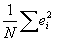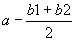Name:_______________________

Economics 345 Final

Prof. Bryan Caplan

Fall, 1997

Instructions:

• You have 2 hours, 45 minutes to complete this exam.
• You may use any books, notes, or other materials that you wish, but avoid spending too much time on any one question.
• Partial credit may be awarded on all questions.
• The maximum possible number of points is 300.
• You should have 10 pages, counting this one.

Part 1: True, False, and Explain

(10 points each - 3 for the right answer, and 7 for the explanation)

State whether each of the following propositions is true or false. Using 2-3 sentences AND/OR equations, explain your answer.

1. You get data on economic growth for four randomly selected countries:
 Country Growth Rate U.S. 2% Britain 3% Japan 1% Mexico 4%

T, F, and Explain: The estimated population variance is 1.25.

2. T, F, and Explain: The covariance between the dependent variable and the error terms is equal to.

3. You regress Y on X, getting the equation: Y=1.237 + .847*X.

T, F, and Explain: Therefore, if you regress Y on ln(X), the equation will be of the form Y=constant + 2.33*ln(X). (Note that e.847=2.33).

4. You have a dependent variable with N observations.

T, F, and Explain: You can always get an R2=1 if you regress the dependent variable on any N variables (including the constant).

5. T, F, and Explain: R2 always increases when you add additional variables to a given regression specification.

6. You do two regressions: in both cases, your dependent variable is cancer severity (on a scale of 1-10; higher is worse), and the independent variable is a dummy variable equal to 1 if the patient received a new drug, and 0 otherwise. Your results are as follows:

Regression #1: Severityi=3+4*Drugi

Regression #2: Severityi=3-2*Drugi

T, F, and Explain: One plausible explanation for the difference is that patients were randomly selected for Regression #1, but self-selected for Regression #2.

1. Education is the number of years a person went to school; Grad is a dummy variable equal to 1 if a person graduated from college, and 0 otherwise. You do the following regression on 1000 employed individuals:

(\$2000) (\$200) (\$1000)

T, F, and Explain: The typical annual salary benefit of completing the final year of college was about \$8000.

Problems #8 and #9 refer to the following regression:

Snails can be either male, female, or hermaphrodite - and they must be one of the three. You run the following regression on a randomly selected box of 10,000 snails to estimate their lifespan (in days):

Lifespani=a + b1*Malei + b2*Femalei

8. T, F, and Explain: It will be possible to run this regression on your computer.

9. T, F, and Explain: The predicted lifespan of hermaphrodite snails will be.

Problems #10 and #11 refer to the following regression:

Unemploymentt=5 -.6*Inflationt +.8*Inflationt-1 +.5*Unemploymentt-1.

10. T, F, and Explain: The equation is consistent with the simple version of the Phillips curve.

11. T, F, and Explain: The implied estimate of the natural rate of unemployment is 5%.

1. Landsburg's piece on "New, Improved Football" discusses an economist's flawed model for predicting the down that teams will punt on.
2. T, F, and Explain: The economist's model could be fixed if he added a new independent variable, TOTALDOWNS to his list of regressors, where TOTALDOWNS is defined as the total number of downs a team gets.

Problems #13 and #14 refer to the following regressions:

(A) NPER= 3 + .9*M2PER + .3*(G1PER) +.3*(G2PER)

(1) (.2) (.1) (.1)

(B) RPER= 1 + .1*M2PER + .3*(G1PER) -.3*(G2PER)

(1) (.2) (.1) (.1)

NPER=percentage change in nominal GDP; RPER is the percentage change in real GDP; M2PER is the percentage change in M2; G1PER is the percentage change in the unpredictable part of government spending - such as military spending; and G2PER is the percentage change in the predictable part of government spending, such as Social Security and Medicare.

3. T, F, and Explain: Equation (A) is consistent with a constant velocity of money.
4.

5. T, F, and Explain: Equation (B) is consistent with the existence of an expectations-augmented Phillips curve.
6.

7. Suppose you regress the percentage change in the oil price today on the percentages changes of: the natural gas price today, the oil price yesterday, and yesterday's federal funds rate.

T, F, and Explain: If the random walk hypothesis holds for the price of oil, then none of the coefficients on these three independent variables will be significantly different from zero.

16. Suppose you define the variable INDEX=1 if a fund is an index fund, and 0 if it is a managed fund. Then you regress the funds' gross ROR (gross rate of return) and net ROR (gross ROR minus management fee) on INDEX:

 Gross RORi=10 + .2*INDEXi (3) (.5) Net RORi=7 +2.2*INDEXi (3) (.5)

T, F, and Explain: Even though managed funds have higher management fees than index funds, this suggests that management fees "pay for themselves" with a higher return.

17. Black markets, though difficult to measure, are usually thought to be much larger in more tightly regulated and heavily taxes economies. GDP figures, however, do not count black market output.

T, F, and Explain: Adding black market output to legal output would probably make the positive correlation between economic freedom and prosperity bigger.

18. T, F, and Explain: According to Sachs' article, rich countries with bad economic policies will grow more slowly than poor countries with similar policies.

(20 points each)

In 4-6 sentences AND/OR equations, answer all of the following questions.

1. PROVE: The residuals in a regression necessarily sum to zero.

2. GIVEN: Y=10 +3*X1 has an R2 of .7.

PROVE: The regression Y=a+b1*X1+b2*X2 will have an R2 of at least .7.

3. Both inflation and unemployment in the U.S. are currently very low. What does the evidence on the expectations-augmented Phillips curve suggest is likely to happen over the next 3 years?

1. Is there any evidence (from class or your homeworks) that government spending affects nominal GDP?
2. "If the random walk hypothesis is correct, then stock prices are totally unpredictable." Evaluate with reference to Landsburg's article "Random Walks and Stock Market Prices."

6. Compare the 1995 Economic Freedom rating of the U.S. to the rating of any other country of your choice. What specific components of the index contribute most to the disparity? Is there any reason to think that these components are caused by prosperity rather than causes of prosperity?